# Convert improper fractions to a mixed number

Any improper fraction can be presented in the form of natural numbers or the sum of a natural number and a proper fraction: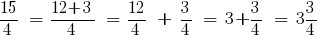## To convert improper fractions to a mixed number, you must:

1. divide the numerator to its denominator;
2. the remainder from dividing the record in the numerator, the denominator remain unchanged;
3. the result of the division to record a part.

### Examples convert the improper fractions to a mixed number

Example 1: to Convert an improper fraction (highlight the full piece):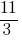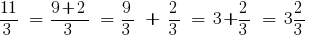The integer part is 3 and the remainder 2

Answer: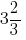Example 2: to Convert an improper fraction (highlight the full piece):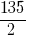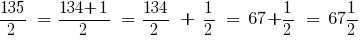The integer part is equal to 67, and the remainder 1

Answer:Example 3: to Convert an improper fraction (highlight the full piece):The integer part is equal to 13, and the remainder 2

Answer: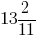## Converting fractions to decimal

In practice, often use decimals, but when a problem is encountered and common and decimal fractions, you should go for one type of fractions (translate decimal fractions in the ordinary or ordinary in decimal). Not always ordinary fraction can be converted to decimal, so the decimal translate.

When translating decimal fractions in the usual in the numerator of the fraction write the number
what is after the decimal, and the bit unit in the denominator (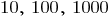and so on) contains the same number of zeros as digits after the decimal point in decimal fractions.

Example 4: Translate a common fraction into a decimal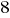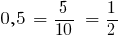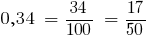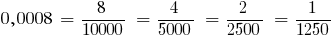If the decimal contains a integer part, convert it into a mixed number and a fractional part of the record before: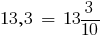Tags:
Chapter:
Versions in other languages:
Share with friends: